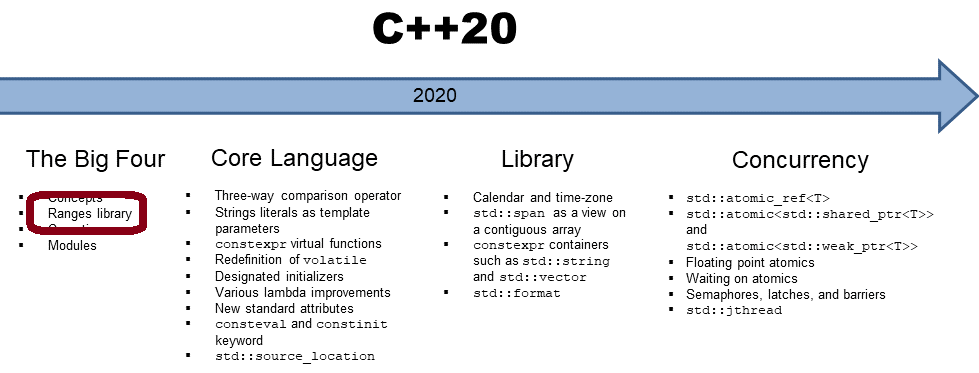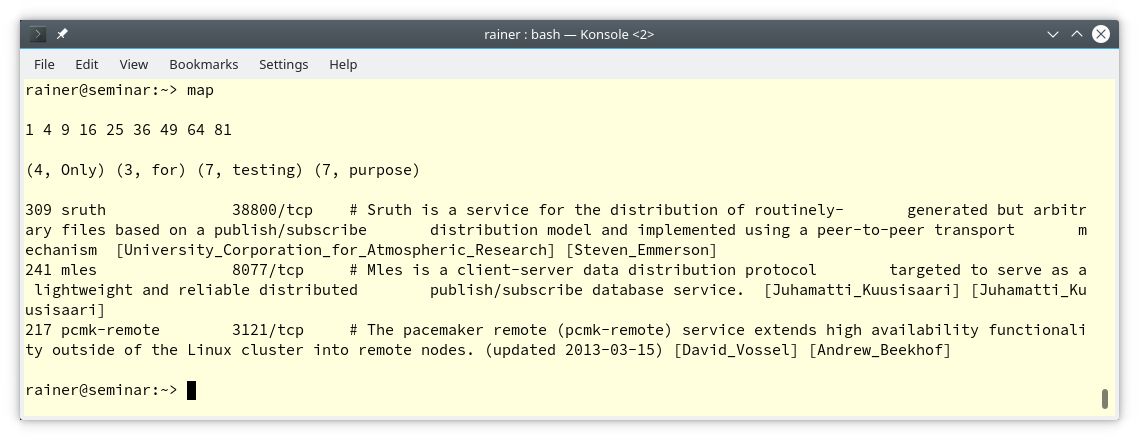# C++20: Python’s map Function

Today, I finish my experiment writing beloved Python functions in C++. So far, I have implemented the Python functions filter, range, and xrange. Today, I have a closer look at the map function and combine the functions map and filter into one function.It was implemented in my last post, “C++20: Pythons range Function, the Second” a lazy variant of range: xrange. A few of my German readers complain that xrange does not behave, such as Python 2 xrange function. My xrange function requires constant expressions for the created numbers’ beginning and end.

```auto res = xrange<1, 10>();
for (auto i: res) std::cout << i << " ";  // 1 2 3 4 5 6 7 8 9
```

In the example, 1 and 10 are constant expressions. This means an expression such as the following one would not compile.

```int begin = 1;
int end = 10;

auto res = xrange<begin, end>();
```

I assume you know what that means?

## Pythons range Function, the Third

Thanks to my German reader Clocktown, I can present the final version of xrange. The function xrange is lazy and can also accept arguments for the boundaries, which are not constant expressions.

```// xrange2.hpp

#include <stdio.h>
#include <range/v3/all.hpp>

namespace view = ranges::views;

auto xrange(long long begin, long long end, long long step = 1) {
if(step == 0) {
throw std::invalid_argument("Step cannot be 0");
}
auto Step = begin < end ? step : -step;
auto Begin = std::min(begin, end);
auto End = Step < 0 ? Begin : std::max(begin, end);
return view::iota(Begin, End)
| view::stride(std::abs(Step))
| view::transform([begin, end](std::size_t i){
return begin < end ? i : end - (i - begin);
});
}

auto xrange(long long end) {
return xrange(0, end, 1);
}
```

The key idea of his implementation is that view::transform eventually transforms the calculation into a reverse variant. xrange can be invoked with one, two, or three arguments. The default for the first argument is 0, and for the third argument is 1. Let’s try it out. I replaced the xrange implementation of my last post with this new implementation.

##Modernes C++ Mentoring

Be part of my mentoring programs:

• "Fundamentals for C++ Professionals" (open)
• "Design Patterns and Architectural Patterns with C++" (open)
• "C++20: Get the Details" (reopens December 2023)
• Do you want to stay informed about my mentoring programs: Subscribe via E-Mail.

```// range2.cpp

#include "xrange2.hpp"

#include <iostream>
#include <range/v3/all.hpp>
#include <vector>

int main() {

std::cout << std::endl;

auto res = xrange(1, 10);
for (auto i: res) std::cout << i << " ";

std::cout << "\n\n";

res = xrange(1, 50, 5);
for (auto i: res) std::cout << i << " ";

std::cout << "\n\n";

auto res2 = xrange(20, 10, -1);
for (auto i: res2) std::cout << i << " ";

std::cout << "\n\n";

res2 = xrange(50, 10, -5);
for (auto i: res2) std::cout << i << " ";

std::cout << "\n\n";

res = xrange(1, 1'000'000'000'000'000'000);
// for (auto i: res) std::cout << i << " ";

for (auto i: res | ranges::views::take(10)) std::cout << i << " ";

std::cout << "\n\n";

for (auto i: res | ranges::views::drop_while([](int i){ return i < 1'000'000; })
| ranges::views::take_while([](int i){ return i < 1'000'010; })){
std::cout << i << " ";
}

std::cout << "\n\n";

}
```

As expected, I got the same result.So far, nothing new. But here are the new use cases. begin and end are not constant expressions, and xrange supports one argument.

```int main() {

int begin = 3;
int end = 7;

for(auto x: xrange(end)) {
std::cout << x << " ";  // 0 1 2 3 4 5 6
}

for(auto x: xrange(begin, end)) {
std::cout << x << " ";  // 3 4 5 6

for(auto x: xrange(end, begin, -2)) {
std::cout << x << " ";  // 7 5
}

}
```

Now, I’m done with the function range and xrange. Let me continue with the function map.

## map

First, here is my simplified definition of Pythons 2 map function. I restrict map to one sequence

• map(function, sequence): Returns a list by applying the function to each input sequence element.

If you think about it, there is one challenge to overcome. In contrast to Pythons function filter (C++20: Pythonic with the Ranges Library), map can change the type of the input sequence.

```// map.cpp

#include "range.hpp"

#include <algorithm>
#include <fstream>
#include <functional>
#include <iostream>
#include <range/v3/all.hpp>
#include <string>
#include <vector>
#include <utility>

template <typename Func, typename Seq>
auto map(Func func, Seq seq) {

typedef typename Seq::value_type value_type;
using return_type = decltype(func(std::declval<value_type>()));     // (4)

std::vector<return_type> result{};
for (auto i :seq | ranges::views::transform(func)) result.push_back(i);

return result;
}

int main() {

std::cout << std::endl;

// map(lambda i: i * i, range(1, 10))                               // (1)
auto res = map([](int i){ return i * i; }, range(1, 10) );

for (auto v: res) std::cout << v << " ";

std::cout << "\n\n";
// (2)
// map(lambda word: (len(word), word), ["Only", "for", "testing", "purpose."])
std::vector<std::string> myStrings{"Only", "for", "testing", "purpose"};
auto res2 = map([](const std::string& s){ return std::make_pair(s.size(), s); }, myStrings);

for (auto p: res2) std::cout << "(" <<  p.first << ", " << p.second << ") " ;

std::cout << "\n\n";
// (3)
// freqWord = map(lambda word: (len(word), word), open("/etc/services").read().split("\n"))
// freqWord.sort(reverse = True)
// freqWord[:3]
std::ifstream file("/etc/services", std::ios::in);
std::stringstream buffer;
buffer << file.rdbuf();
std::string text = buffer.str();

std::vector<std::string> words = text | ranges::views::split('\n');   // (4)
auto lengthWords = map([](const std::string& s){ return std::make_pair(s.size(), s); }, words);
std::sort(std::begin(lengthWords), std::end(lengthWords), std::greater);
std::for_each(std::begin(lengthWords), std::begin(lengthWords) + 3, [](const auto& p) {
std::cout << p.first << " " << p.second << std::endl;
});

std::cout << std::endl;

}
```

Line (4) deduces the return_type. The return_type is the type to which all input sequence elements are transformed if the function func is applied to them. std::declval<value_type>() returns a rvalue reference which can be used by decltype to deduce the type.

The commented-out lines are the corresponding Python code.

1. maps each element to its square
2. maps each word to a pair length of the word, and the word
3. Reads each line from the file “/etc/services“, maps each line to the pair length of the line and the line, sorts the resulting sequence in reverse order, and displays the 3 longest lines.

The screenshot shows the output of the program.I almost forgot to mention another issue I had to implement the map function. The call std::vector words = text | ranges::views::split(‘\n’); (line 4) is deprecated. Instead, I should use the conversion operator ranges::to. ranges::to is not part of C++20, so I asked the author of the ranges library, Eric Niebler, what I should do. He proposed a quite wordy solution that triggered a GCC bug.  Here is the bug report 93936 from Eric. Finally, I stick to the deprecated version.

The function map is not the end of my experiments. I said to myself. Let’s combine map and filter into one function and create something similar to list comprehension in C++. Honestly, I’m not 100% satisfied with the result.

## A flavor of list comprehension

My function mapFilter can only handle one sequence in contrast to list comprehension in Python.

```// mapFilter.cpp

#include "range.hpp"

#include <algorithm>
#include <cctype>
#include <fstream>
#include <functional>
#include <iostream>
#include <range/v3/all.hpp>
#include <string>
#include <vector>
#include <utility>

template <typename T>
struct AlwaysTrue {                        // (1)
constexpr bool operator()(const T&) const {
return true;
}
};
// (2)
template <typename Map, typename Seq, typename Filt = AlwaysTrue<typename Seq::value_type>>
auto mapFilter(Map map, Seq seq, Filt filt = Filt()) {

typedef typename Seq::value_type value_type;
using return_type = decltype(map(std::declval<value_type>()));

std::vector<return_type> result{};
for (auto i :seq | ranges::views::filter(filt)
| ranges::views::transform(map)) result.push_back(i);
return result;
}

int main() {

std::cout << std::endl;
// (3)
// [ i * i for i in range(1, 10) ]
auto res = mapFilter([](int i){ return i * i; }, range(1, 10) );

// (4)
// [ i * i for i in range(1, 10) if i % 2 == 1 ]
res = mapFilter([](int i){ return i * i; }, range(1, 10) ,
[](auto i){ return i % 2 == 1; });

for (auto v: res) std::cout << v << " ";

std::cout << "\n\n";

// (3)
// [(len(word), word) for word in ["Only", "for", "testing", "purpose."]]
std::vector<std::string> myStrings{"Only", "for", "testing", "purpose"};
auto res2 = mapFilter([](const std::string& s){ return std::make_pair(s.size(), s); }, myStrings);

// (5)
// [(len(word), word) for word in ["Only", "for", "testing", "purpose."] if word.isupper()]
myStrings = {"Only", "for", "testing", "purpose"};
res2 = mapFilter([](const std::string& s){ return std::make_pair(s.size(), s); }, myStrings,
[](const std::string& word){ return std::isupper(word); });

for (auto p: res2) std::cout << "(" <<  p.first << ", " << p.second << ") " ;

std::cout << "\n\n";

// (3)
// freqWord = [(len(line), line) for line in open("/etc/services").read().split("\n")]
// freqWord = map(lambda word: (len(word), word), open("/etc/services").read().split("\n"))
// freqWord.sort(reverse = True)
// freqWord[:3]
std::ifstream file("/etc/services", std::ios::in);
std::stringstream buffer;
buffer << file.rdbuf();
std::string text = buffer.str();

std::vector<std::string> words = text | ranges::views::split('\n');
auto lengthWords = mapFilter([](const std::string& s){ return std::make_pair(s.size(), s); }, words);
std::sort(std::begin(lengthWords), std::end(lengthWords), std::greater());

// (6)
// len([line for line in open("/etc/services").read().split("\n") if 100 < len(line) < 150])
words = text | ranges::views::split('\n');
auto allLines = mapFilter([](const std::string& line){ return line; }, words,
[](const std::string& line){ return 100 < line.size() && line.size() < 150; });
std::cout << "Number of lines: " << allLines.size();

std::cout << "\n\n";
}
```

The default predicate that the filter function applies (line 2) consistently returns true (line 1). Always true means that the function mapFilter behaves per default, such as the map function. When you study all lines numbered (3), you see no difference to the previous program map.cpp. But now, the difference begins. The corresponding list comprehensions in Python are commented out.

• Line (4) calculates the square of the odd numbers.
• Line (5) returns pairs (length of the word, word) if the word starts with a capital character.
• Line (6) returns a vector of all lines of the file “/etc/services“, which have between 100 and 150 characters.

## What’s next?

This post was a little longer than usual. My next post is about generalized functions that can be paused and resumed. To make it short: my next post is about coroutines.

Thanks a lot to my Patreon Supporters: Matt Braun, Roman Postanciuc, Tobias Zindl, G Prvulovic, Reinhold Dröge, Abernitzke, Frank Grimm, Sakib, Broeserl, António Pina, Sergey Agafyin, Андрей Бурмистров, Jake, GS, Lawton Shoemake, Jozo Leko, John Breland, Venkat Nandam, Jose Francisco, Douglas Tinkham, Kuchlong Kuchlong, Robert Blanch, Truels Wissneth, Kris Kafka, Mario Luoni, Friedrich Huber, lennonli, Pramod Tikare Muralidhara, Peter Ware, Daniel Hufschläger, Alessandro Pezzato, Bob Perry, Satish Vangipuram, Andi Ireland, Richard Ohnemus, Michael Dunsky, Leo Goodstadt, John Wiederhirn, Yacob Cohen-Arazi, Florian Tischler, Robin Furness, Michael Young, Holger Detering, Bernd Mühlhaus, Matthieu Bolt, Stephen Kelley, Kyle Dean, Tusar Palauri, Dmitry Farberov, Juan Dent, George Liao, Daniel Ceperley, Jon T Hess, Stephen Totten, Wolfgang Fütterer, Matthias Grün, Phillip Diekmann, Ben Atakora, Ann Shatoff, Rob North, Bhavith C Achar, and Marco Parri Empoli.

Thanks, in particular, to Jon Hess, Lakshman, Christian Wittenhorst, Sherhy Pyton, Dendi Suhubdy, Sudhakar Belagurusamy, Richard Sargeant, Rusty Fleming, John Nebel, Mipko, Alicja Kaminska, Slavko Radman, and David Poole.

 My special thanks to EmbarcaderoMy special thanks to PVS-StudioMy special thanks to Tipi.buildMy special thanks to Take Up Code## Seminars

I’m happy to give online seminars or face-to-face seminars worldwide. Please call me if you have any questions.

### Standard Seminars (English/German)

Here is a compilation of my standard seminars. These seminars are only meant to give you a first orientation.

• C++ – The Core Language
• C++ – The Standard Library
• C++ – Compact
• C++11 and C++14
• Concurrency with Modern C++
• Design Pattern and Architectural Pattern with C++
• Embedded Programming with Modern C++
• Generic Programming (Templates) with C++

#### New

• Clean Code with Modern C++
• C++20

### Contact Me

Modernes C++ Mentoring,Tags:
0 replies